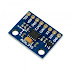The ADXL345 is a complete 3-axis acceleration measurement system with a selectable measurement range of ±2 g, ±4 g, ±8 g, or ±16 g. It measures both dynamic acceleration resulting from motion or shock and static acceleration, such as gravity, which allows the device to be used as a tilt sensor. In this tutorial we are going to interface ADXL345 with STM32.
ADXL345 can be used for a whole bunch of things for eg-
• To measure acceleration in three axis
• To measure Roll and Pitch
• To measure single or double tap
etc..
Today in this tutorial, we will only cover the measurement of acceleration in three axis. And rest of them will be covered in the near future.

## CONNECTIONS

ADXL345 requires 2.0 to 3.6 v so we will give it the typical 3.3v from the microcontroller. I will be using I2C to connect it as I prefer I2C over SPI. You can check the  connections below:-

## INITIALISATION

In order to initialise ADXL345, we need to modify POWER_CTL Register (0x2D) and DATA_FORMAT Register (0x31).
First RESET all bits of POWER_CTL register by writing 0 to them.
Next SET the MEASURE bit, RESET the SLEEP bit and SET the frequency in the WAKE UP bits

Next, in the DATA_FORMAT Register, Set the RANGE using D0 and D1.

``````adxl_write (0x2d, 0x00);  // reset all bits
adxl_write (0x31, 0x01);  // data_format range= +- 4g
adxl_write (0x2d, 0x08);  // measure and wake up 8hz
``````
Before writing the code, just make sure that everything is alright by checking the DEVID of the device. The DEVID register (0x00)  holds a fixed device ID code of 0xE5 . If the code matches 0xE5, we can proceed further.

Now if the ID is matched, we can go ahead and read the data and display it on the screen.
The data is stored in the Registers 0x32 to 0x37 in the form of DATAX0, DATAX1, DATAY0, DATAY1, DATAZ0, DATAZ1. Each Register stores 8 bits of data So let’s see how to read it using I2C.
``````void adxl_read_values (uint8_t reg)
{
}
``````

We are performing Mem_Read because we are reading from specific memory address i.e. 0x32 and we have to read 6 bytes of data from this address onward. Now we need to convert the DATAX0, DATAX1 into single data and this can be done by
``````adxl_read_values (0x32);
x = ((data_rec<<8)|data_rec);
y = ((data_rec<<8)|data_rec);
z = ((data_rec<<8)|data_rec);``````
I get the following result while reading the RAW data in the X, Y and Z axis without any calibration.

Now we need to convert this data into the g form in order to check for the acceleration in specific axis. As you can check above in the initialisation part that I have set the range of ±4 g. According to datasheet, for the range of ±4 g, the scale factor is 7.8mg/LSB.

So to convert into g, we need to multiply with .0078
``````xg = x * .0078;
yg = y * .0078;
zg = z * .0078;``````
This is it Now we can display this data on the display. Here I am using OLED display and I already wrote a tutorial about interfacing OLED with STM32. You can check it HERE.
To display the data on the OLED I wrote the following functions

``````void display_data (float data)
{
sprintf (x_char, "% 4f", data);
SSD1306_Puts (x_char, &Font_11x18, 1);
SSD1306_UpdateScreen ();  // update display
}``````

and
``````   SSD1306_GotoXY (16, 1);
SSD1306_Puts ("X: ", &Font_11x18, 1);
SSD1306_GotoXY (16, 20);
SSD1306_Puts ("Y: ", &Font_11x18, 1);
SSD1306_GotoXY (32, 20);
display_data (yg);
SSD1306_GotoXY (16, 40);
SSD1306_Puts ("Z: ", &Font_11x18, 1);
SSD1306_GotoXY (32, 40);
display_data (zg);
SSD1306_GotoXY (32, 1);
display_data (xg);
``````
ADXL345 Accelerometer using STM32Reviewed by arun singh on March 13, 2018 Rating: 5